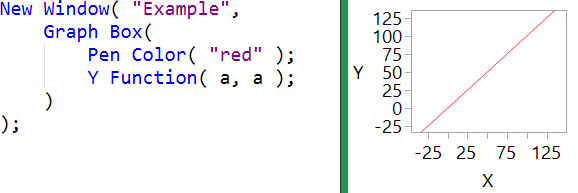World Statistics Day was yesterday, but we’re celebrating all week long! This celebration means acknowledging the impact statistics has on our world. Who is your favorite statistician? Share with us who they are and why they top your favorites list.
Choose Language Hide Translation Bar
Highlighted

## GraphBuilder Customization in JSL: Draw Line Segment DYnamically = Error "Invalid Matrix Token"

Hi JMP Community;

In my quest to automate some of the more tedious tasks of annotation Graph Builder plots, I'm trying to dynamically add horizontal line segments between data groups using the following script. Unfortunately, it looks like I cannot use simple Expr () followed by Substitute (), and Eval () for this specific task. Note: I'm working with sensitive data therefore I will need to create mock DATA and STAT tables if necessary.

``````Names Default to Here (1);

dt1 = Data Table ("DATA.JMP");
dt2 = Data Table ("STAT.JMP");

gbe = Expr (dt1 << Graph Builder(
Size( 699, 625 ),
Variables(
X( :R vs NR ),
Y( _YS_ ),
Color( :R vs NR )
),
Elements(
Points( X, Y, Legend( 6 ) ),
Box Plot( X, Y, Legend( 8 ), Outliers( 0 ) )
),
SendToReport(

Dispatch(
{},
"Graph Builder",
FrameBox,
2,
Description( "Script" ),
Pen Size (3);

// The following line of code return the error "Invalid Matrix Token"
Line ([0.2,0.8], [Y1, Y1]);
Text( Center Justified, {0.5, top1}, VAL1 );

// The following line of code return the error "Invalid Matrix Token"
Line ([0.2,1.8], [Y2, Y2]);
Text( Center Justified, {1.0, top2}, VAL2 );

// The following line of code return the error "Invalid Matrix Token"
Line ([1.2,1.8], [Y1, Y1]);
Text( Center Justified, {1.5, top1}, VAL3 );
), Grid Line Order( 1 ), Reference Line Order( 3 )}
)
)
)
);

For (i = 749, i <= 750, i++,

YCOL = Column (dt1, i);
PVAL1 = "p VAL = " ||  CHAR(format(Column (dt2, 2) [i-747],"PValue"));
PVAL2 = "p VAL = " ||  CHAR(format(Column (dt2, 3) [i-747],"PValue"));
PVAL3 = "p VAL = " ||  CHAR(format(Column (dt2, 4) [i-747],"PValue"));

data_topy1 = Col Quantile (YCOL, 0.99);
data_topy2 = Col Quantile (YCOL, 0.975);

gbx = Substitute(Name Expr (gbe),
Expr (_YS_), YCOL,
Expr (VAL1), PVAL1,
Expr (VAL2), PVAL2,
Expr (VAL3), PVAL3,
Expr (Y1), data_topy1,
Expr (Y2), data_topy2
);

gb = Eval (gbx);

gb << journal;
gb << close window;

);``````

Any ideas would be welcome.

Thank you

Thierry R. Sornasse
1 ACCEPTED SOLUTION

Accepted Solutions
Highlighted

## Re: GraphBuilder Customization in JSL: Draw Line Segment DYnamically = Error "Invalid Matrix Token"

Your solution is a great one for this case since HLine and VLine are infinitely long. Here's an explanation and some alternatives.

The square bracket matrix is a compile-time constant and can't use variables. I've often used concatenation operators to build small matrices like this:

``````a = 17;
b = 23;
Show( a || b, a |/ b );``````

a || b = [17 23];
a |/ b = [17, 23];

You can also use matrix():

``matrix( {{a, b}, {a, a}} );``

[17 23, 17 17]

If your line needed to be bounded at 0.2 and 1.8, you could use a different form of line that takes lists rather than matrices:

``line( {x1,y1}, {x2,y2} )``

The line function evaluates the expressions in each list.

And, if you needed an infinitely long line at some angle other than horizontal or vertical, you could use the YFunction():YFunction

Craige
2 REPLIES 2
Highlighted

## Re: GraphBuilder Customization in JSL: Draw Line Segment DYnamically = Error "Invalid Matrix Token"

Hi,

I found a work around: instead of calling the Line() function, I replaced it with H Line () which does not require coordinates as a matrix.

Best regards,

TS
Thierry R. Sornasse
Highlighted

## Re: GraphBuilder Customization in JSL: Draw Line Segment DYnamically = Error "Invalid Matrix Token"

Your solution is a great one for this case since HLine and VLine are infinitely long. Here's an explanation and some alternatives.

The square bracket matrix is a compile-time constant and can't use variables. I've often used concatenation operators to build small matrices like this:

``````a = 17;
b = 23;
Show( a || b, a |/ b );``````

a || b = [17 23];
a |/ b = [17, 23];

You can also use matrix():

``matrix( {{a, b}, {a, a}} );``

[17 23, 17 17]

If your line needed to be bounded at 0.2 and 1.8, you could use a different form of line that takes lists rather than matrices:

``line( {x1,y1}, {x2,y2} )``

The line function evaluates the expressions in each list.

And, if you needed an infinitely long line at some angle other than horizontal or vertical, you could use the YFunction():YFunction

Craige
Article Labels

There are no labels assigned to this post.